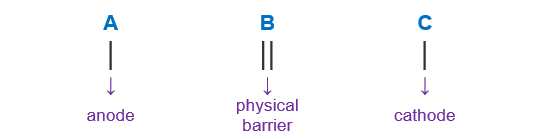Problem: Use line notation to represent each electrochemical cell 2 Ag+(aq) + Pb(s) → 2 Ag (s) +Pb2+(aq)

FREE Expert Solution

We’re being asked to determine the cell notation for the redox reaction shown below:

2 Ag+(aq) + Pb(s) → 2 Ag (s) +Pb2+(aq)

When writing a cell notation, we use the following format – “as easy as ABCTo determine the cell notation of the reaction, we will use the following steps:

Step 1: Separate the reaction into two half-reactions.
Step 2: Determine the anode and the cathode.
Step 3: Write the cell notation of the reaction.

86% (425 ratings)
Problem Details

Use line notation to represent each electrochemical cell

2 Ag+(aq) + Pb(s) → 2 Ag (s) +Pb2+(aq)